Publicité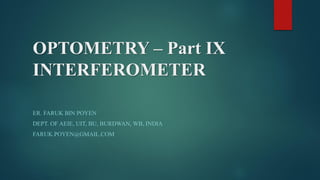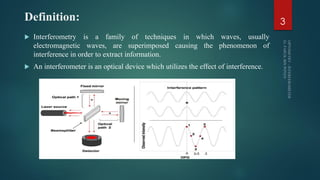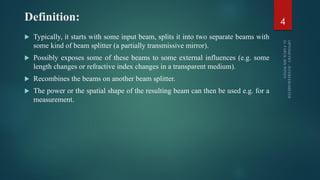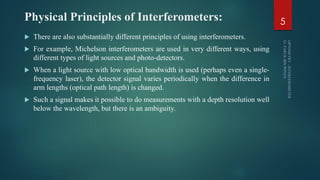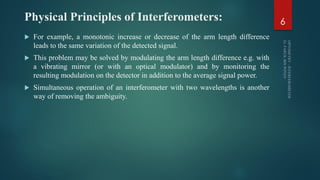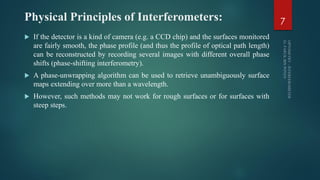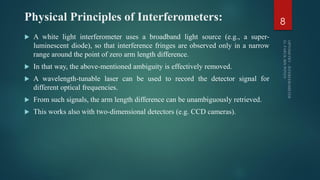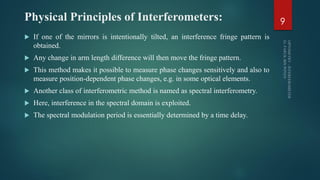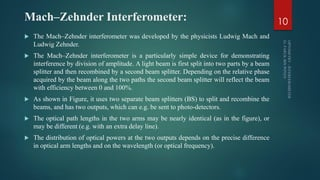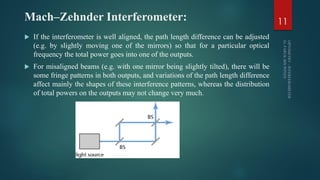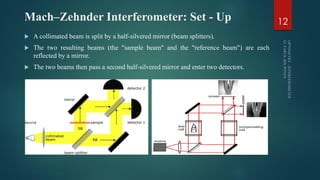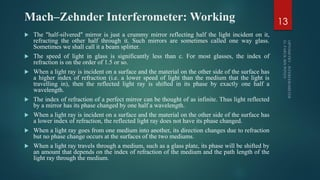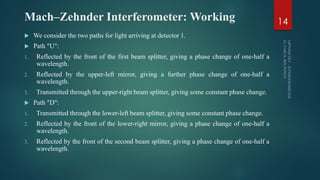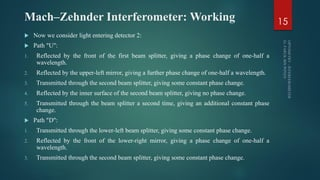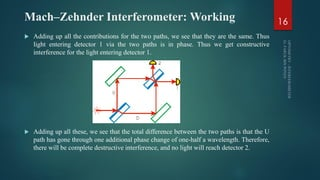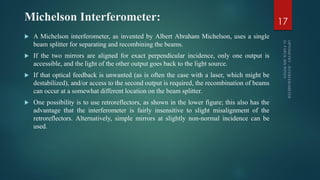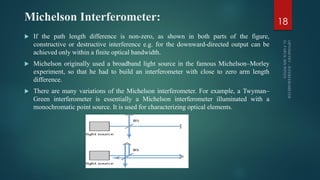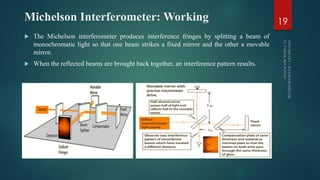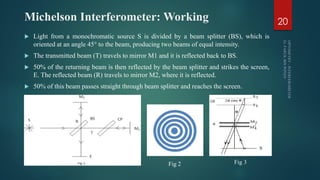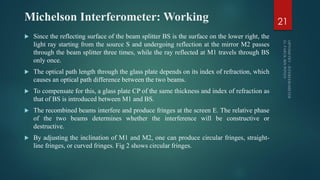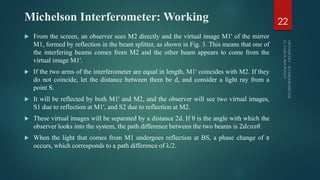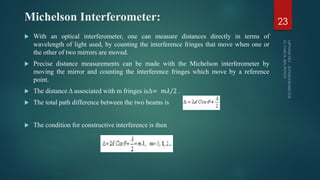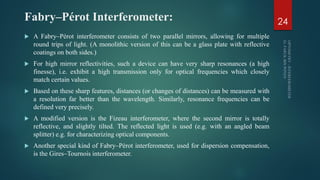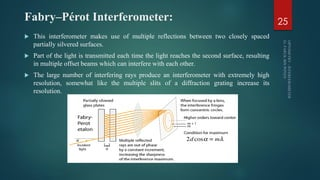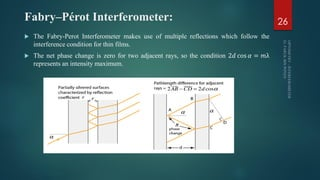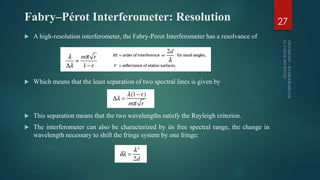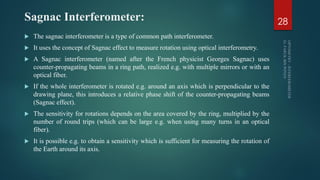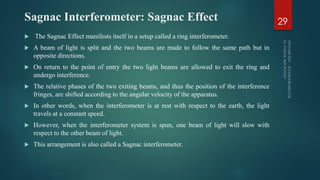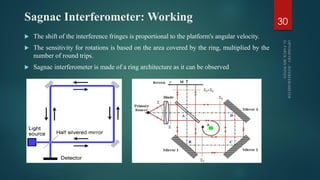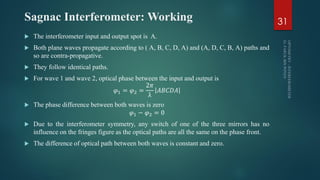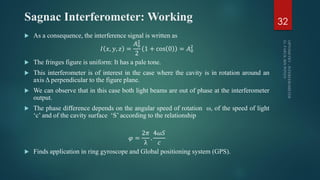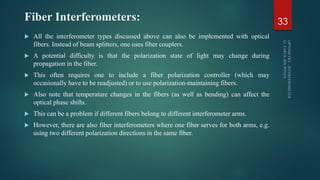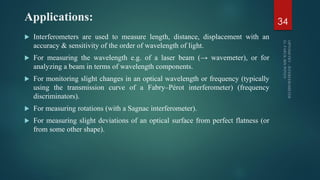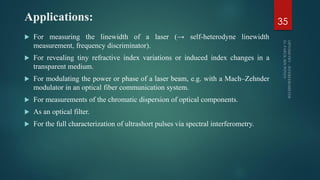1 sur 36
Publicité

### Optical Instrumentation 9. Interferometer

1. OPTOMETRY – Part IX INTERFEROMETER ER. FARUK BIN POYEN DEPT. OF AEIE, UIT, BU, BURDWAN, WB, INDIA FARUK.POYEN@GMAIL.COM
2. Contents:  Definition  Physical Principles of Interferometers  Mach–Zehnder Interferometer  Michelson Interferometer  Fabry–Pérot Interferometer  Sagnac Interferometer  Fiber Interferometers  Applications of Interferometer 2
3. Definition:  Interferometry is a family of techniques in which waves, usually electromagnetic waves, are superimposed causing the phenomenon of interference in order to extract information.  An interferometer is an optical device which utilizes the effect of interference. 3
4. Definition:  Typically, it starts with some input beam, splits it into two separate beams with some kind of beam splitter (a partially transmissive mirror).  Possibly exposes some of these beams to some external influences (e.g. some length changes or refractive index changes in a transparent medium).  Recombines the beams on another beam splitter.  The power or the spatial shape of the resulting beam can then be used e.g. for a measurement. 4
5. Physical Principles of Interferometers:  There are also substantially different principles of using interferometers.  For example, Michelson interferometers are used in very different ways, using different types of light sources and photo-detectors.  When a light source with low optical bandwidth is used (perhaps even a single- frequency laser), the detector signal varies periodically when the difference in arm lengths (optical path length) is changed.  Such a signal makes it possible to do measurements with a depth resolution well below the wavelength, but there is an ambiguity. 5
6. Physical Principles of Interferometers:  For example, a monotonic increase or decrease of the arm length difference leads to the same variation of the detected signal.  This problem may be solved by modulating the arm length difference e.g. with a vibrating mirror (or with an optical modulator) and by monitoring the resulting modulation on the detector in addition to the average signal power.  Simultaneous operation of an interferometer with two wavelengths is another way of removing the ambiguity. 6
7. Physical Principles of Interferometers:  If the detector is a kind of camera (e.g. a CCD chip) and the surfaces monitored are fairly smooth, the phase profile (and thus the profile of optical path length) can be reconstructed by recording several images with different overall phase shifts (phase-shifting interferometry).  A phase-unwrapping algorithm can be used to retrieve unambiguously surface maps extending over more than a wavelength.  However, such methods may not work for rough surfaces or for surfaces with steep steps. 7
8. Physical Principles of Interferometers:  A white light interferometer uses a broadband light source (e.g., a super- luminescent diode), so that interference fringes are observed only in a narrow range around the point of zero arm length difference.  In that way, the above-mentioned ambiguity is effectively removed.  A wavelength-tunable laser can be used to record the detector signal for different optical frequencies.  From such signals, the arm length difference can be unambiguously retrieved.  This works also with two-dimensional detectors (e.g. CCD cameras). 8
9. Physical Principles of Interferometers:  If one of the mirrors is intentionally tilted, an interference fringe pattern is obtained.  Any change in arm length difference will then move the fringe pattern.  This method makes it possible to measure phase changes sensitively and also to measure position-dependent phase changes, e.g. in some optical elements.  Another class of interferometric method is named as spectral interferometry.  Here, interference in the spectral domain is exploited.  The spectral modulation period is essentially determined by a time delay. 9
10. Mach–Zehnder Interferometer:  The Mach–Zehnder interferometer was developed by the physicists Ludwig Mach and Ludwig Zehnder.  The Mach–Zehnder interferometer is a particularly simple device for demonstrating interference by division of amplitude. A light beam is first split into two parts by a beam splitter and then recombined by a second beam splitter. Depending on the relative phase acquired by the beam along the two paths the second beam splitter will reflect the beam with efficiency between 0 and 100%.  As shown in Figure, it uses two separate beam splitters (BS) to split and recombine the beams, and has two outputs, which can e.g. be sent to photo-detectors.  The optical path lengths in the two arms may be nearly identical (as in the figure), or may be different (e.g. with an extra delay line).  The distribution of optical powers at the two outputs depends on the precise difference in optical arm lengths and on the wavelength (or optical frequency). 10
11. Mach–Zehnder Interferometer:  If the interferometer is well aligned, the path length difference can be adjusted (e.g. by slightly moving one of the mirrors) so that for a particular optical frequency the total power goes into one of the outputs.  For misaligned beams (e.g. with one mirror being slightly tilted), there will be some fringe patterns in both outputs, and variations of the path length difference affect mainly the shapes of these interference patterns, whereas the distribution of total powers on the outputs may not change very much. 11
12. Mach–Zehnder Interferometer: Set - Up  A collimated beam is split by a half-silvered mirror (beam splitters).  The two resulting beams (the "sample beam" and the "reference beam") are each reflected by a mirror.  The two beams then pass a second half-silvered mirror and enter two detectors. 12
13. Mach–Zehnder Interferometer: Working  The "half-silvered" mirror is just a crummy mirror reflecting half the light incident on it, refracting the other half through it. Such mirrors are sometimes called one way glass. Sometimes we shall call it a beam splitter.  The speed of light in glass is significantly less than c. For most glasses, the index of refraction is on the order of 1.5 or so.  When a light ray is incident on a surface and the material on the other side of the surface has a higher index of refraction (i.e. a lower speed of light than the medium that the light is travelling in), then the reflected light ray is shifted in its phase by exactly one half a wavelength.  The index of refraction of a perfect mirror can be thought of as infinite. Thus light reflected by a mirror has its phase changed by one half a wavelength.  When a light ray is incident on a surface and the material on the other side of the surface has a lower index of refraction, the reflected light ray does not have its phase changed.  When a light ray goes from one medium into another, its direction changes due to refraction but no phase change occurs at the surfaces of the two mediums.  When a light ray travels through a medium, such as a glass plate, its phase will be shifted by an amount that depends on the index of refraction of the medium and the path length of the light ray through the medium. 13
14. Mach–Zehnder Interferometer: Working  We consider the two paths for light arriving at detector 1.  Path "U": 1. Reflected by the front of the first beam splitter, giving a phase change of one-half a wavelength. 2. Reflected by the upper-left mirror, giving a further phase change of one-half a wavelength. 3. Transmitted through the upper-right beam splitter, giving some constant phase change.  Path "D": 1. Transmitted through the lower-left beam splitter, giving some constant phase change. 2. Reflected by the front of the lower-right mirror, giving a phase change of one-half a wavelength. 3. Reflected by the front of the second beam splitter, giving a phase change of one-half a wavelength. 14
15. Mach–Zehnder Interferometer: Working  Now we consider light entering detector 2:  Path "U": 1. Reflected by the front of the first beam splitter, giving a phase change of one-half a wavelength. 2. Reflected by the upper-left mirror, giving a further phase change of one-half a wavelength. 3. Transmitted through the second beam splitter, giving some constant phase change. 4. Reflected by the inner surface of the second beam splitter, giving no phase change. 5. Transmitted through the beam splitter a second time, giving an additional constant phase change.  Path "D": 1. Transmitted through the lower-left beam splitter, giving some constant phase change. 2. Reflected by the front of the lower-right mirror, giving a phase change of one-half a wavelength. 3. Transmitted through the second beam splitter, giving some constant phase change. 15
16. Mach–Zehnder Interferometer: Working  Adding up all the contributions for the two paths, we see that they are the same. Thus light entering detector 1 via the two paths is in phase. Thus we get constructive interference for the light entering detector 1.  Adding up all these, we see that the total difference between the two paths is that the U path has gone through one additional phase change of one-half a wavelength. Therefore, there will be complete destructive interference, and no light will reach detector 2. 16
17. Michelson Interferometer:  A Michelson interferometer, as invented by Albert Abraham Michelson, uses a single beam splitter for separating and recombining the beams.  If the two mirrors are aligned for exact perpendicular incidence, only one output is accessible, and the light of the other output goes back to the light source.  If that optical feedback is unwanted (as is often the case with a laser, which might be destabilized), and/or access to the second output is required, the recombination of beams can occur at a somewhat different location on the beam splitter.  One possibility is to use retroreflectors, as shown in the lower figure; this also has the advantage that the interferometer is fairly insensitive to slight misalignment of the retroreflectors. Alternatively, simple mirrors at slightly non-normal incidence can be used. 17
18. Michelson Interferometer:  If the path length difference is non-zero, as shown in both parts of the figure, constructive or destructive interference e.g. for the downward-directed output can be achieved only within a finite optical bandwidth.  Michelson originally used a broadband light source in the famous Michelson–Morley experiment, so that he had to build an interferometer with close to zero arm length difference.  There are many variations of the Michelson interferometer. For example, a Twyman– Green interferometer is essentially a Michelson interferometer illuminated with a monochromatic point source. It is used for characterizing optical elements. 18
19. Michelson Interferometer: Working  The Michelson interferometer produces interference fringes by splitting a beam of monochromatic light so that one beam strikes a fixed mirror and the other a movable mirror.  When the reflected beams are brought back together, an interference pattern results. 19
20. Michelson Interferometer: Working  Light from a monochromatic source S is divided by a beam splitter (BS), which is oriented at an angle 45° to the beam, producing two beams of equal intensity.  The transmitted beam (T) travels to mirror M1 and it is reflected back to BS.  50% of the returning beam is then reflected by the beam splitter and strikes the screen, E. The reflected beam (R) travels to mirror M2, where it is reflected.  50% of this beam passes straight through beam splitter and reaches the screen. 20 Fig 2 Fig 3
21. Michelson Interferometer: Working  Since the reflecting surface of the beam splitter BS is the surface on the lower right, the light ray starting from the source S and undergoing reflection at the mirror M2 passes through the beam splitter three times, while the ray reflected at M1 travels through BS only once.  The optical path length through the glass plate depends on its index of refraction, which causes an optical path difference between the two beams.  To compensate for this, a glass plate CP of the same thickness and index of refraction as that of BS is introduced between M1 and BS.  The recombined beams interfere and produce fringes at the screen E. The relative phase of the two beams determines whether the interference will be constructive or destructive.  By adjusting the inclination of M1 and M2, one can produce circular fringes, straight- line fringes, or curved fringes. Fig 2 shows circular fringes. 21
22. Michelson Interferometer: Working  From the screen, an observer sees M2 directly and the virtual image M1' of the mirror M1, formed by reflection in the beam splitter, as shown in Fig. 3. This means that one of the interfering beams comes from M2 and the other beam appears to come from the virtual image M1'.  If the two arms of the interferometer are equal in length, M1' coincides with M2. If they do not coincide, let the distance between them be d, and consider a light ray from a point S.  It will be reflected by both M1' and M2, and the observer will see two virtual images, S1 due to reflection at M1', and S2 due to reflection at M2.  These virtual images will be separated by a distance 2d. If θ is the angle with which the observer looks into the system, the path difference between the two beams is 2𝑑𝑐𝑜𝑠𝜃.  When the light that comes from M1 undergoes reflection at BS, a phase change of π occurs, which corresponds to a path difference of λ/2. 22
23. Michelson Interferometer:  With an optical interferometer, one can measure distances directly in terms of wavelength of light used, by counting the interference fringes that move when one or the other of two mirrors are moved.  Precise distance measurements can be made with the Michelson interferometer by moving the mirror and counting the interference fringes which move by a reference point.  The distance Δ associated with m fringes isΔ= 𝑚𝜆/2 .  The total path difference between the two beams is  The condition for constructive interference is then 23
24. Fabry–Pérot Interferometer:  A Fabry–Pérot interferometer consists of two parallel mirrors, allowing for multiple round trips of light. (A monolithic version of this can be a glass plate with reflective coatings on both sides.)  For high mirror reflectivities, such a device can have very sharp resonances (a high finesse), i.e. exhibit a high transmission only for optical frequencies which closely match certain values.  Based on these sharp features, distances (or changes of distances) can be measured with a resolution far better than the wavelength. Similarly, resonance frequencies can be defined very precisely.  A modified version is the Fizeau interferometer, where the second mirror is totally reflective, and slightly tilted. The reflected light is used (e.g. with an angled beam splitter) e.g. for characterizing optical components.  Another special kind of Fabry–Pérot interferometer, used for dispersion compensation, is the Gires–Tournois interferometer. 24
25. Fabry–Pérot Interferometer:  This interferometer makes use of multiple reflections between two closely spaced partially silvered surfaces.  Part of the light is transmitted each time the light reaches the second surface, resulting in multiple offset beams which can interfere with each other.  The large number of interfering rays produce an interferometer with extremely high resolution, somewhat like the multiple slits of a diffraction grating increase its resolution. 25
26. Fabry–Pérot Interferometer:  The Fabry-Perot Interferometer makes use of multiple reflections which follow the interference condition for thin films.  The net phase change is zero for two adjacent rays, so the condition 2𝑑 cos 𝛼 = 𝑚λ represents an intensity maximum. 26
27. Fabry–Pérot Interferometer: Resolution  A high-resolution interferometer, the Fabry-Perot Interferometer has a resolvance of  Which means that the least separation of two spectral lines is given by  This separation means that the two wavelengths satisfy the Rayleigh criterion.  The interferometer can also be characterized by its free spectral range, the change in wavelength necessary to shift the fringe system by one fringe: 27
28. Sagnac Interferometer:  The sagnac interferometer is a type of common path interferometer.  It uses the concept of Sagnac effect to measure rotation using optical interferometry.  A Sagnac interferometer (named after the French physicist Georges Sagnac) uses counter-propagating beams in a ring path, realized e.g. with multiple mirrors or with an optical fiber.  If the whole interferometer is rotated e.g. around an axis which is perpendicular to the drawing plane, this introduces a relative phase shift of the counter-propagating beams (Sagnac effect).  The sensitivity for rotations depends on the area covered by the ring, multiplied by the number of round trips (which can be large e.g. when using many turns in an optical fiber).  It is possible e.g. to obtain a sensitivity which is sufficient for measuring the rotation of the Earth around its axis. 28
29. Sagnac Interferometer: Sagnac Effect  The Sagnac Effect manifests itself in a setup called a ring interferometer.  A beam of light is split and the two beams are made to follow the same path but in opposite directions.  On return to the point of entry the two light beams are allowed to exit the ring and undergo interference.  The relative phases of the two exiting beams, and thus the position of the interference fringes, are shifted according to the angular velocity of the apparatus.  In other words, when the interferometer is at rest with respect to the earth, the light travels at a constant speed.  However, when the interferometer system is spun, one beam of light will slow with respect to the other beam of light.  This arrangement is also called a Sagnac interferometer. 29
30. Sagnac Interferometer: Working  The shift of the interference fringes is proportional to the platform's angular velocity.  The sensitivity for rotations is based on the area covered by the ring, multiplied by the number of round trips.  Sagnac interferometer is made of a ring architecture as it can be observed 30
31. Sagnac Interferometer: Working  The interferometer input and output spot is A.  Both plane waves propagate according to ( A, B, C, D, A) and (A, D, C, B, A) paths and so are contra-propagative.  They follow identical paths.  For wave 1 and wave 2, optical phase between the input and output is 𝜑1 = 𝜑2 = 2𝜋 λ 𝐴𝐵𝐶𝐷𝐴  The phase difference between both waves is zero 𝜑1 − 𝜑2 = 0  Due to the interferometer symmetry, any switch of one of the three mirrors has no influence on the fringes figure as the optical paths are all the same on the phase front.  The difference of optical path between both waves is constant and zero. 31
32. Sagnac Interferometer: Working  As a consequence, the interference signal is written as 𝐼 𝑥, 𝑦, 𝑧 = 𝐴0 2 2 1 + cos 0 = 𝐴0 2  The fringes figure is uniform: It has a pale tone.  This interferometer is of interest in the case where the cavity is in rotation around an axis Δ perpendicular to the figure plane.  We can observe that in this case both light beams are out of phase at the interferometer output.  The phase difference depends on the angular speed of rotation ω, of the speed of light ‘c’ and of the cavity surface ‘S’ according to the relationship 𝜑 = 2𝜋 λ . 4𝜔𝑆 𝑐  Finds application in ring gyroscope and Global positioning system (GPS). 32
33. Fiber Interferometers:  All the interferometer types discussed above can also be implemented with optical fibers. Instead of beam splitters, one uses fiber couplers.  A potential difficulty is that the polarization state of light may change during propagation in the fiber.  This often requires one to include a fiber polarization controller (which may occasionally have to be readjusted) or to use polarization-maintaining fibers.  Also note that temperature changes in the fibers (as well as bending) can affect the optical phase shifts.  This can be a problem if different fibers belong to different interferometer arms.  However, there are also fiber interferometers where one fiber serves for both arms, e.g. using two different polarization directions in the same fiber. 33
34. Applications:  Interferometers are used to measure length, distance, displacement with an accuracy & sensitivity of the order of wavelength of light.  For measuring the wavelength e.g. of a laser beam (→ wavemeter), or for analyzing a beam in terms of wavelength components.  For monitoring slight changes in an optical wavelength or frequency (typically using the transmission curve of a Fabry–Pérot interferometer) (frequency discriminators).  For measuring rotations (with a Sagnac interferometer).  For measuring slight deviations of an optical surface from perfect flatness (or from some other shape). 34
35. Applications:  For measuring the linewidth of a laser (→ self-heterodyne linewidth measurement, frequency discriminator).  For revealing tiny refractive index variations or induced index changes in a transparent medium.  For modulating the power or phase of a laser beam, e.g. with a Mach–Zehnder modulator in an optical fiber communication system.  For measurements of the chromatic dispersion of optical components.  As an optical filter.  For the full characterization of ultrashort pulses via spectral interferometry. 35
36. References:  www.rp-photonics.com/interferometers.html  https://en.wikipedia.org/wiki/Mach%E2%80%93Zehnder_interferometer  https://www.cs.princeton.edu/courses/archive/fall06/cos576/papers/zetie_et_al_mach_ze hnder00.pdf  http://hyperphysics.phy-astr.gsu.edu/hbase/phyopt/michel.html  http://vlab.amrita.edu/?sub=1&brch=189&sim=1106&cnt=1  http://hyperphysics.phy-astr.gsu.edu/hbase/phyopt/fabry.html#c1  https://en.wikipedia.org/wiki/Sagnac_effect  http://www.optique-ingenieur.org/en/courses/OPI_ang_M02_C05/co/Contenu_26.html 36
Publicité